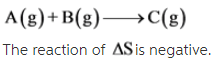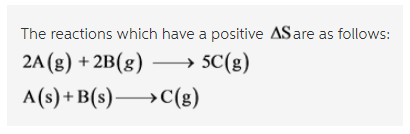# Which of the following reactions have a positive ?Srxn? Check all that apply?

Without referring to a data table, rank the following compounds by standard molar entropy.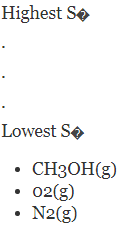Which of the following reactions have a positive ?Srxn? Check all that apply.

2A(g) + B(g) --> C(g)
2A(g) + 2B (g) --> 5C (g)
A(s) + B(s) --> C(g)
A(g) + B(g) --> C(g)

Concepts and reason
The standard molar entropy describes the entropy content of one mole of substance under standard pressure and temperature.

It also describes the randomness and disorders of molecules, depending on the number of various arrangements possible for the molecules.

Fundamentals
As the randomness of the molecules increases, the entropy also increases and gives a positive sign.

Conditions for to be positive:

1.Entropy increases as the phase changes from solid to liquid, liquid to gas or solid to gas.
2.If the change in the number of moles is positive, then the entropy is also positive.

The increasing order of entropy is as follows: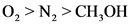The given reaction is as follows: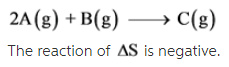The given reaction is as follows: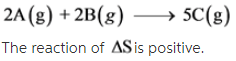The given reaction is as follows: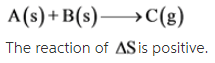The given reaction is as follows: